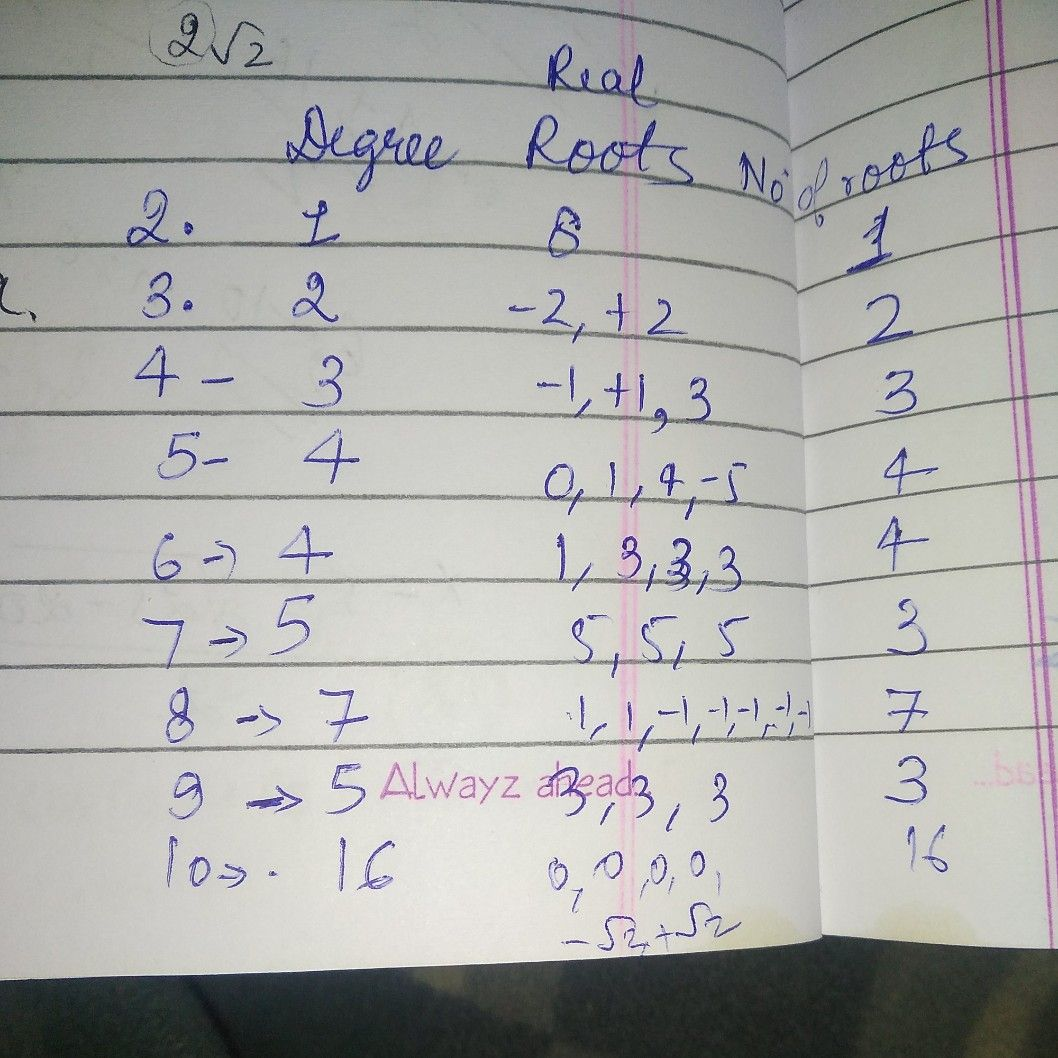Symbol
ProblemActivity 2: Finding the Number of Roots of Polynomial Equations Some polynomial equations are given below. Complete the table and answer the questions that follow. (If a root occurs twice, count it twice; if thrice, count it three times, and $s0$ on. The first 'one is done for you) Polynomial Equation Degree Real Roots of Number of an Equation Real Roots $,1$ $\left(x+1\right)^{2}\left(x-5\right)=0$ $3$ $-1$ $\left(2$ times); 5 $2.x-8=0$ $3$ $\left(x+2\right)\left(x-2\right)=0$ $4$ $\left(x-3\right)\left(x+1\right)\left(x-1\right)=0$ $5.$ $x\left(x-4\right)\left(x+5\right)\left(x-1\right)=0$ $6$ $\left(x-1\right)\left(x-3\right)^{3}=0$ $7.\left(x^{2}-4x+13\right)\left(x-5\right)^{3}=0$ $8.$ $\left(x+1\right)^{5}\left(x-1\right)^{2}=0$ $9.\left(x^{2}+4\right)\left(x-3\right)^{3}=0$ $10.\left(x-\sqrt{2} \right)^{6}\left(x+\sqrt{2} \right)^{6}x^{4}=0$